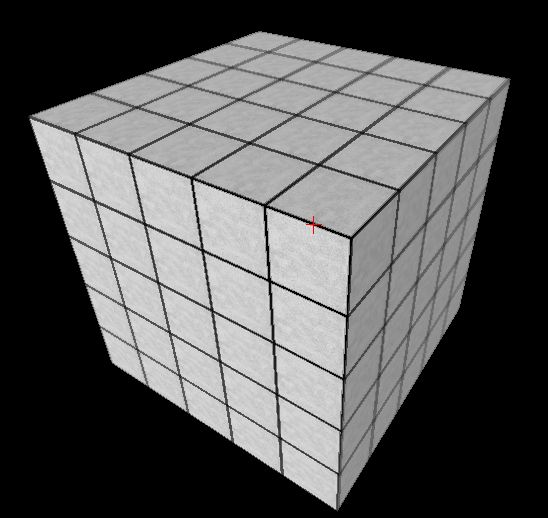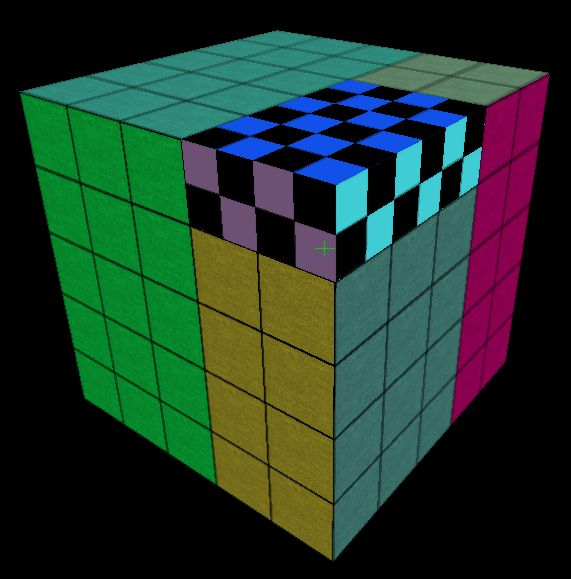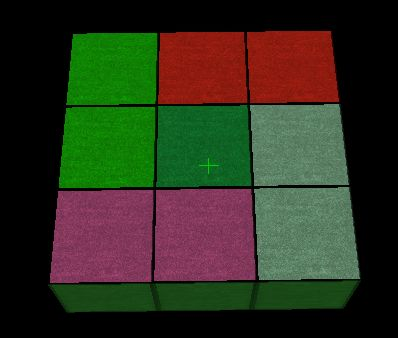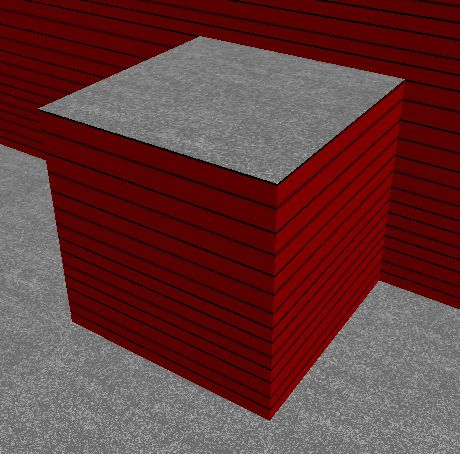Home --> Programming Projects --> Block Engine --> Block + Quad Algorithm
The Block Algorithm is a technique used in virtually every "Block Engine", but the Quad Algorithm is not. The Block Algorithm is used to iteratively compute the surface 1x1 squares of the scene. The Quad Algorithm is used to iteratively merge the 1x1 squares, computed by the Block Algorithm, into N by M quads. These algorithms significantly decrease the amount of geometry that needs to be rendered while still alowing blocks to be created and deleted in real time. This page describes these algorithms.

# Rendering Block Worlds

The first obstacle is to render large block levels efficiently. For now, let us ignore lighting. We want an algorithm to render the scene quickly that still allows the player to create and destroy blocks (and thus incrementally change the world).

Consider an array of 5x5x5 blocks:The simplest algorithm to render this would be to render each of the six sides of each individual cube. That is, 5*5*5*6 = 750 quads would be sent to the graphics card. Ouch! A more efficient approach is to render only the 1x1 squares that are "on the outside". If this was done, then only 5*5*6 = 150 quads would be sent to the graphics card. A data structure to calculate which squares are on the outside would works as follows: keep track of all squares that would be drawn using the naive algorithm, sort these by position and facing direction, and "cancel out" (but not delete) squares that are in the same position but have faces in the opposite direction. This data structure can be incrementally changed as the world changes. Let us call this the Block Algorithm.

We can do much better than 150 quads. We can do this by "merging" adjacent 1x1 squares that are on the same plane and have the same texture to form a single rectangular quad. Doing this in the above example, only 6 quads would need to be sent to the graphics card! That is much better than the naive algorithm that uses 750 quads! Let us call this algorithm (of merging 1x1 squares together that have the same texture, are on the same plane, and are facing the same direction) the Quad Algorithm.

The following is an example of the quad algorithm in effect, where different quads are colored randomly for demonstration:The quad algorithm is a bit of a pain to implement, but the overall idea is simple. A data structure of all merged quads needs to be maintained, were quads are sorted by plane (and normal direction) and texture. The API for the Quad Algorithm consists of two functions: 1) add a 1x1 square and 2) remove a 1x1 square. The Block Algorithm calls the "add a 1x1 square" function when a 1x1 square becomes visible. The Block Algorithm calls the "remove a 1x1 square" function when a 1x1 square becomes invisible. The implementation of the Quad Algorithm (for a particular plane and texture combination) works as follows (AddQuad and RemoveQuad are helper funcitons):
2. RemoveSquare(x):
find the quad y that contains x,
split y into subquad pieces where x is one such piece,
remove y from the data structure,
and call AddQuad(p) for each piece p of y other than x.
Determine if x can be merged with any other quad y.
If not, add x to the data structure.
Remove the quad x from the data structure.
Why is this a pain to implement? There are three basic reasons:
1. A data structure, such as a 2D-interval tree, would need to be used to efficiently perform the step of determining which quad contains a particular square.
2. To determine if one quad can be merged to another quad efficiently, quads need to be queried by their edges.
3. When breaking up a quad into smaller pieces (at most 5 subquads), there are various cases which makes this tedious to program.
The algorithm is greedy, and it is possible for more quads to be used than is theoretically necessary. This can happen if blocks are added to the world in an irregular order. This problem occurs only to a minor extent in practice. Here is an example of this phenomenon:Note: the memory used by the Quad Algorithm is proportional to the numer of quads, not the number of 1x1 squares! The Block Algorithm, on the other hand, needs to maintain this information.

# Improving the Block Algorithm

As mentioned above, the block algorithm keeps track of each individual block. However, this is not necessary. All that is needed is a mechonism to determine the contents of any given position in the world. This could be done procedurally, or the block information could be compressed somehow. Once a block C is either added to or removed from the world, the "Add Square" or "Remove Square" procedures of the Quad Algorithm could be called according to the textures of the blocks adjacent to C. All that matters is that the Quad Algorithm is originally in a consistent state.

# Changing An Individual SideWe have been treating blocks as if they have the same texture on every side, but of course this is not necessary. We could have a system where the texture of each side of a block (top, bottom, front, back, left, right) is determined by its type. See, for example, the above picture. Another thing we might what to do is manually change the texture of one side of a block (as if we were putting on wallpaper). We might want to do this to make the walls of the outside of an indoor map invisible so we do not waste time rendering them (this process is described here). It is clear how to change the interface of the Block Algorithm to accomodate this change.

# The Quad Algorithm and Chunking

It is a natural idea to break the block world up into chunks (say, of dimension 16 x 16 x 16). One reason to do this is so that an entire chunk can be rendered atomically using, say, a Vertex Buffer Object. Also, view frustrum culling can be used to avoid rendering chunks that are outside the view frustrum. These are both extremely desirable features.

Unfortunately, there is a slight clash between the idea of chunking and the Quad Algorithm. That is, if we want to use chunks in the ways described above, then every quad must be contained completely in a chunk. To accomplish this, the Quad Algorithm would be performed for each chunk. What is annoying is that more quads may be needed to render a scene than is theoretically necessary. This is problematic in the case that the block would to be rendered is extremely large but also quite homogeneous, so only a few quads are needed in theory. It is possible that I am the only person bothered by this issue.

If one is devoted to the idea of using the quad algorithm globally and not have any chunks, then the problem of view frustrum culling becomes quite a headache. Determining whether each individual quad is outside the view frustrum will take too long, so multiple quads need to be considered at once. Conceivably, one could impose some data structure on the collection of quads to speed up this procedure, but this will likely be more trouble than it is worth.

Since chunking is so essential, it seems like the best idea is to perform the quad algorithm within each chunk and not worry about how the chunking may theoretically limit the scenes that can be rendered.

# Culling and Chunking

Suppose we have broken the block world into chunks of dimension 16 by 16 by 16. If we know which chunk contains the camera, we can use a trick to prevent many quads from being rendered. That is, there are only 6 directions that any quad can be facing. We can sort the quads within each chunk based on which direction they are facing. Then, based on the relative position of the camera's chunk and the chunk to be rendered, we can quickly determine which sides might have quads that will not be back-faced culled by the graphics card. This is one of the beautiful benefits of rendering block worlds instead of more general scenes.